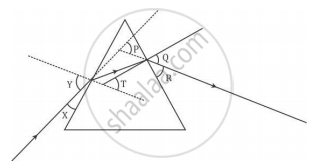# The Angle of Emergence and the Angle of Deviation in Digram Are - Science

In the following diagram, the path of a ray of light passing through a glass prism is shown:In this diagram the angle of incidence, the angle of emergence and the angle of deviation respectively are (select the correct option):

(A) X, R and T

(B) Y, Q and T

(C) X, Q and P

(D) Y, Q and P

#### Solution

The angle made by the incident ray with the normal to the first face of the prism is called incident angle, which is angle Y here.

The angle made by the emergent ray with the normal to the surface when it comes out from the prism after refraction is called emergent angle, which is angle Q here.

The angle between the incident ray and the emergent ray is called angle of deviation, which is angle P here.

Hence, the correct option is D.

Concept: Dispersion of Light Through Prism and Formation of Spectrum
Is there an error in this question or solution?